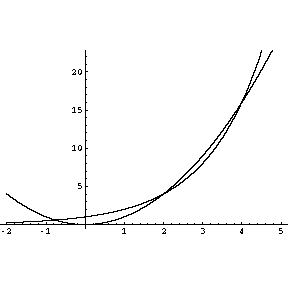my name is john Question for calculus For what values of x is 2 to the exponent x greater than x squared? Hi John, I would first try to solve 2x = x2. Two solutions are x = 2 and x = 4 and a plot of the two functions shows that there is another solution at approximately x = -0.8.Cheers, Harley Go to Math Central